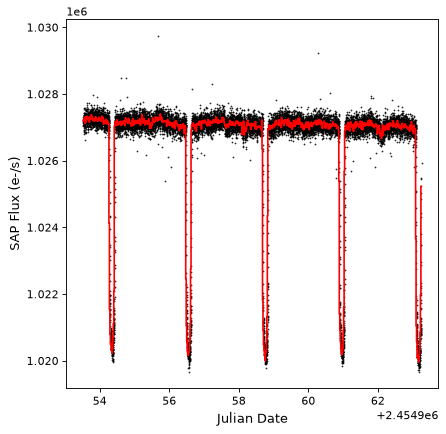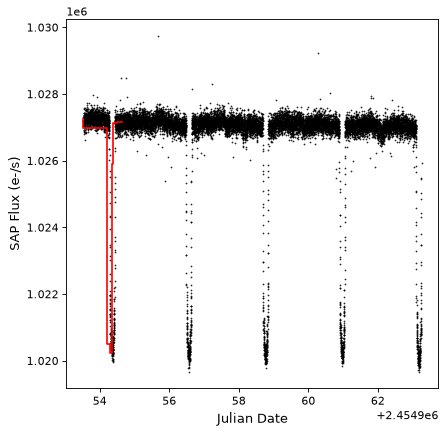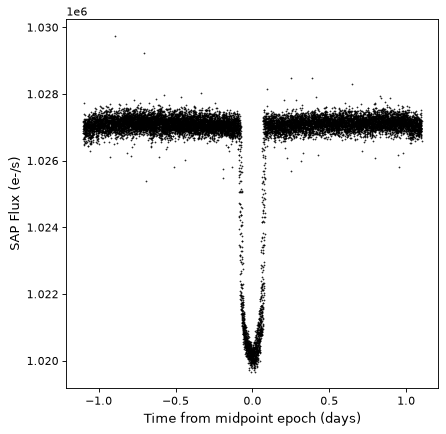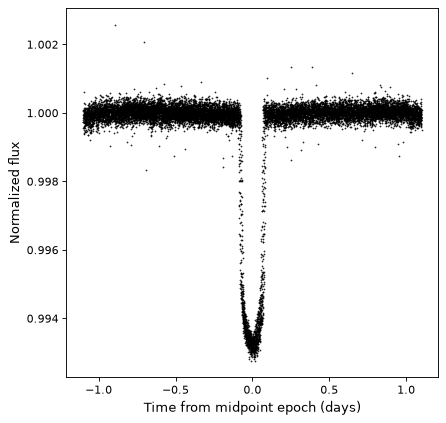# Manipulation and Analysis of Time Series¶

## Combining Time Series¶

The `vstack()` and `hstack()` functions from the `astropy.table` module can be used to stack time series in different ways.

### Examples¶

Time series can be stacked “vertically” or row-wise using the `vstack()` function (although note that sampled time series cannot be combined with binned time series and vice versa):

```>>> from astropy.table import vstack
>>> from astropy import units as u
>>> from astropy.timeseries import TimeSeries
>>> ts_a = TimeSeries(time_start='2016-03-22T12:30:31',
...                   time_delta=3 * u.s,
...                   data={'flux': [1, 4, 5, 3, 2] * u.mJy})
>>> ts_b = TimeSeries(time_start='2016-03-22T12:50:31',
...                   time_delta=3 * u.s,
...                   data={'flux': [4, 3, 1, 2, 3] * u.mJy})
>>> ts_ab = vstack([ts_a, ts_b])
>>> ts_ab
<TimeSeries length=10>
time            flux
mJy
Time          float64
----------------------- -------
2016-03-22T12:30:31.000     1.0
2016-03-22T12:30:34.000     4.0
2016-03-22T12:30:37.000     5.0
2016-03-22T12:30:40.000     3.0
2016-03-22T12:30:43.000     2.0
2016-03-22T12:50:31.000     4.0
2016-03-22T12:50:34.000     3.0
2016-03-22T12:50:37.000     1.0
2016-03-22T12:50:40.000     2.0
2016-03-22T12:50:43.000     3.0
```

Note that `vstack()` does not automatically sort, nor get rid of duplicates — this is something you would need to do explicitly afterwards.

Time series can also be combined “horizontally” or column-wise with other tables using the `hstack()` function, though these should not be time series (as having multiple time columns would be confusing):

```>>> from astropy.table import Table, hstack
>>> data = Table(data={'temperature': [40., 41., 40., 39., 30.] * u.K})
>>> ts_a_data = hstack([ts_a, data])
>>> ts_a_data
<TimeSeries length=5>
time            flux  temperature
mJy          K
Time          float64    float64
----------------------- ------- -----------
2016-03-22T12:30:31.000     1.0        40.0
2016-03-22T12:30:34.000     4.0        41.0
2016-03-22T12:30:37.000     5.0        40.0
2016-03-22T12:30:40.000     3.0        39.0
2016-03-22T12:30:43.000     2.0        30.0
```

## Sorting Time Series¶

Sorting time series in place can be done using the `sort()` method, as for `Table`:

```>>> ts = TimeSeries(time_start='2016-03-22T12:30:31',
...                 time_delta=3 * u.s,
...                 data={'flux': [1., 4., 5., 3., 2.]})
>>> ts
<TimeSeries length=5>
time            flux
Time          float64
----------------------- -------
2016-03-22T12:30:31.000     1.0
2016-03-22T12:30:34.000     4.0
2016-03-22T12:30:37.000     5.0
2016-03-22T12:30:40.000     3.0
2016-03-22T12:30:43.000     2.0
>>> ts.sort('flux')
>>> ts
<TimeSeries length=5>
time            flux
Time          float64
----------------------- -------
2016-03-22T12:30:31.000     1.0
2016-03-22T12:30:43.000     2.0
2016-03-22T12:30:40.000     3.0
2016-03-22T12:30:34.000     4.0
2016-03-22T12:30:37.000     5.0
```

## Resampling¶

We provide a `aggregate_downsample()` function that can be used to bin values from a time series into equal-size or uneven bins, and contiguous and non-contiguous bins, using a custom function (mean, median, etc.). This operation returns a `BinnedTimeSeries`. Note that this is a basic function in the sense that it does not, for example, know how to treat columns with uncertainties differently from other values, and it will blindly apply the custom function specified to all columns.

### Example¶

The following example shows how to use `aggregate_downsample()` to bin a light curve from the Kepler mission into 20 minute contiguous bins using a median function. First, we read in the data using:

```from astropy.timeseries import TimeSeries
from astropy.utils.data import get_pkg_data_filename
example_data = get_pkg_data_filename('timeseries/kplr010666592-2009131110544_slc.fits')
```

(See Reading and Writing Time Series for more details about reading in data). We can then downsample using:

```import numpy as np
from astropy import units as u
from astropy.timeseries import aggregate_downsample
kepler_binned = aggregate_downsample(kepler, time_bin_size=20 * u.min, aggregate_func=np.nanmedian)
```

We can take a look at the results:

```import matplotlib.pyplot as plt
plt.plot(kepler.time.jd, kepler['sap_flux'], 'k.', markersize=1)
plt.plot(kepler_binned.time_bin_start.jd, kepler_binned['sap_flux'], 'r-', drawstyle='steps-pre')
plt.xlabel('Julian Date')
plt.ylabel('SAP Flux (e-/s)')
```

(png, svg, pdf)The `aggregate_downsample()` can also be used to bin the light curve into custom bins. The following example shows the case of uneven-size contiguous bins:

```kepler_binned = aggregate_downsample(kepler, time_bin_size=[1000, 125, 80, 25, 150, 210, 273] * u.min,
aggregate_func=np.nanmedian)

plt.plot(kepler.time.jd, kepler['sap_flux'], 'k.', markersize=1)
plt.plot(kepler_binned.time_bin_start.jd, kepler_binned['sap_flux'], 'r-', drawstyle='steps-pre')
plt.xlabel('Julian Date')
plt.ylabel('SAP Flux (e-/s)')
```

(png, svg, pdf)To learn more about the custom binning functionality in `aggregate_downsample()`, see Initializing a Binned Time Series.

## Folding¶

The `TimeSeries` class has a `fold()` method that can be used to return a new time series with a relative and folded time axis. This method takes the period as a `Quantity`, and optionally takes an epoch as a `Time`, which defines a zero time offset:

```kepler_folded = kepler.fold(period=2.2 * u.day, epoch_time='2009-05-02T20:53:40')

plt.plot(kepler_folded.time.jd, kepler_folded['sap_flux'], 'k.', markersize=1)
plt.xlabel('Time from midpoint epoch (days)')
plt.ylabel('SAP Flux (e-/s)')
```

(png, svg, pdf)Note that in this example we happened to know the period and midpoint from a previous periodogram analysis. See the example in Time Series (astropy.timeseries) for how you might do this.

## Arithmetic¶

Since `TimeSeries` objects are subclasses of `Table`, they naturally support arithmetic on any of the data columns. As an example, we can take the folded Kepler time series we have seen in previous examples, and normalize it to the sigma-clipped median value.

```from astropy.stats import sigma_clipped_stats

mean, median, stddev = sigma_clipped_stats(kepler_folded['sap_flux'])

kepler_folded['sap_flux_norm'] = kepler_folded['sap_flux'] / median

plt.plot(kepler_folded.time.jd, kepler_folded['sap_flux_norm'], 'k.', markersize=1)
plt.xlabel('Time from midpoint epoch (days)')
plt.ylabel('Normalized flux')
```

(png, svg, pdf)